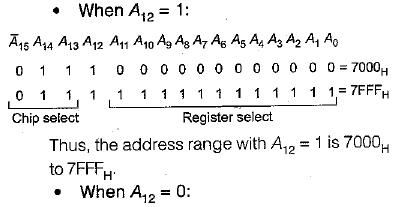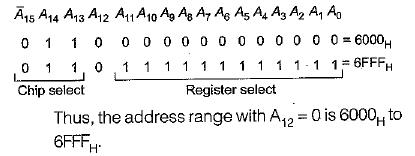Courses

# Microprocessor Interfacing

## 10 Questions MCQ Test Topicwise Question Bank for Electronics Engineering | Microprocessor Interfacing

Description
This mock test of Microprocessor Interfacing for Electronics and Communication Engineering (ECE) helps you for every Electronics and Communication Engineering (ECE) entrance exam. This contains 10 Multiple Choice Questions for Electronics and Communication Engineering (ECE) Microprocessor Interfacing (mcq) to study with solutions a complete question bank. The solved questions answers in this Microprocessor Interfacing quiz give you a good mix of easy questions and tough questions. Electronics and Communication Engineering (ECE) students definitely take this Microprocessor Interfacing exercise for a better result in the exam. You can find other Microprocessor Interfacing extra questions, long questions & short questions for Electronics and Communication Engineering (ECE) on EduRev as well by searching above.
QUESTION: 1

### The number of address lines required to address 8 K bytes of memory is

Solution:

Size of memory= 8 K bytes
= 8 x 1024 x 8 bits
= 213 x 8 bits
= 2n x m bits
Here, n = No. of address lines = 13
and m = No. of data lines = 8

QUESTION: 2

Solution:
QUESTION: 3

### The number of memory chips of size 1 K x 4-bits required to build a memory bank of size 16 K x 8 bits is

Solution:

Size of given chip
= 1 K x 4 bits
= 1024 x 4 bits

Size of required memory bank
= 16 K x 8 bits
= 16 x 1024 x 8 bits

Required number of memory chips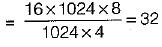QUESTION: 4

The maximum number of memory and I/O devices that can be addressed in a 8085 microprocessor based system is:

Solution:

An 8085 microprocessor has 16 address lines and 8 data lines. Thus, it can address maximum of 216 I/O devices and memory.

QUESTION: 5

The logic circuit used to generate the active low chip select (CS) by an 8085 microprocessor to address a peripheral is shown in figure below.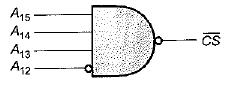The address range for which the device will respond is:

Solution:

For chip select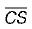signal to be low, we have: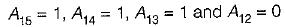The address range with this combination will be as follows: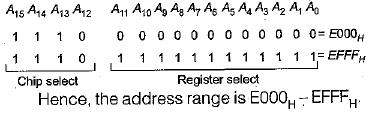QUESTION: 6

The I/O devices in 8085 microprocessor can be used in:

Solution:

In an 8085 microprocessor, the I/O devices can be used is a memory mapped I/O only. An 8085 microprocessor is an 8 – bit microprocessor that is designed by the Intel in 1977 using the NMOS technology.  This 8085 microprocessor has an 8 – bit data bus, a 16 – bit address bus, a 16 – bit program counter, a 16 – bit stack pointer, Six 8-bit registers arranged in pairs like BC, DE, HL. This processor is usually found in mobile phones, washing machines, microwave ovens.

QUESTION: 7

Eight memory chips of size 64 x 8 bits have their address buses connected together. What will be the size of the resultant memory?

Solution:

Size of the given memory chip = 64 x 8 bits = 26 x 8 bits = 2n x m bits

Here, n = 6 and m - 8
Hence, the chip has 6 address lines and 8 data lines.
Now, when eight memory chips of size 26 x 8 bits have their address buses connected together, then the address lines will be same but data lines will become 8 x 8 = 64. Thus, the size of the resultant memory will be 64 x 64 bits

QUESTION: 8

Consider the following statements associated with memory mapped I/O scheme of communication with microprocessor:
1. It reduces the memory space available.
2. Arithmetic or logical operation can be directly performed with I/O data.
3. The processor cannot manipulate I/O data residing in interface registers with the same instructions that are used to manipulate memory location.
4. The processor on treats an interface register as a part of the memory system.

Which of the statements given above is/are not correct?

Solution:

Statement-3 is not correct because in memory mapped I/O scheme of communication with microprocessor, the processor manipulates ‘ registers with same instruction that are used to manipulate memory locations.

QUESTION: 9

An 8-bit microcontroller has an external RAM with the memory map from 8000 H to 9FFF H. The number of bytes this RAM can store is:

Solution:

One memory location of an 8-bit microcontroller external RAM will store 8 bits of data or 1 byte. The number of memory locations in the memory range from 8000 H to 9FFF H are equal to (9FFF - 8000)H + 1 = 2000 H.
Hence, the number of bytes the memory locations from 8000 H to 9FFF H can store is equal to 2000 H or 8192.

QUESTION: 10

What is the address range for 4 K RAM used in 8085 microprocessor if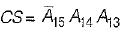's used as the chip select logic?

Solution:

For 4 K RAM (4 x 1024 = 212), the number of address lines required will be 12. Hence, out of the 16 address'lines, 12 addresses lines (i.e. A11., to A0) will be used for addressing the memory location in a chip and the remaining four (i.e. A15 to A12) will be used for selecting the chip. Thus, the chip select signal is CS =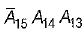.  So, the address lines A15 = 0, A14 = 1 and A13 = 1. The line A12 may be 0 or 1 and hence we will get separate range corresponding to A12 = 1 and A12 = 0